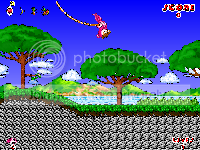-=+=- -=+=- -=+=- -=+=- -=+=- -=+=- -=+=- -=+=- -=+=- -=+=- -=+=- -=+=- -=+=- -=+=- -=+=- -=+=- -=+=- -=+=- -=+=- -=+=- -=+=- -=+=- -=+=- -=+=- -=+=- -=+=- -=+=- -=+=- -=+=- -=+=- (c) WidthPadding Industries 1987 0|373|0 -=+=- -=+=- -=+=- -=+=- -=+=- -=+=- -=+=- -=+=- -=+=- -=+=- -=+=- -=+=- -=+=- -=+=- -=+=- -=+=- -=+=- -=+=- -=+=- -=+=- -=+=- -=+=- -=+=- -=+=- -=+=- -=+=- -=+=- -=+=- -=+=- -=+=- SoCoder -> Showcase Home -> Platformer Games with characters that run and jump. <-- Newer Older -->Blockman in a Mario Costume He's Back! Jayenkai Blitz Max Cross PlatformNeoPlatDS NeonPlat without the Neon! Jayenkai Monkey Nintendo DSJNKPlat 2010 It's another one!! Jayenkai Blitz Max Cross PlatformOperation: Sourcecode A platformer by me, Afr0! Afr0 C/C++ WindowsAvalanche Retro The flash game lives... Flame The Great Monkey WindowsJNKPlat DS 08 Even more JNKPlat!! Jayenkai Basic Cross PlatformFluffy in the enchanted Land omadan Blitz WindowsRetro Raider aka Lara Croft's Cross Quest Jayenkai Blitz Windows Xmas Special 3D platformer jayparker Blitz WindowsBlockman Carry all the blocklets home! Jayenkai Blitz WindowsJNKPlat : DS A brand new JNKPlat puzzle game Jayenkai Basic Cross PlatformNinja Attack Defend Hyphadon from the Gherkins! shroom_monk Blitz WindowsHero (Greenie - v0.9) Remake of the Activision classic Jayenkai Blitz WindowsJNKPlat 07 (0.2) It's JNKPlat! Yeay! Jayenkai Blitz WindowsOnionHead Vendetta (Abandoned) An old, abandoned, 2D platformer game HoboBen Blitz Windows Ball Person Demo demo of my platformer the_mopenator QBasicRetroRaider - Three Crystals Laura Plat heads into the caves once more. Jayenkai , Monkey-X Cross Platform <-- Newer Older -->